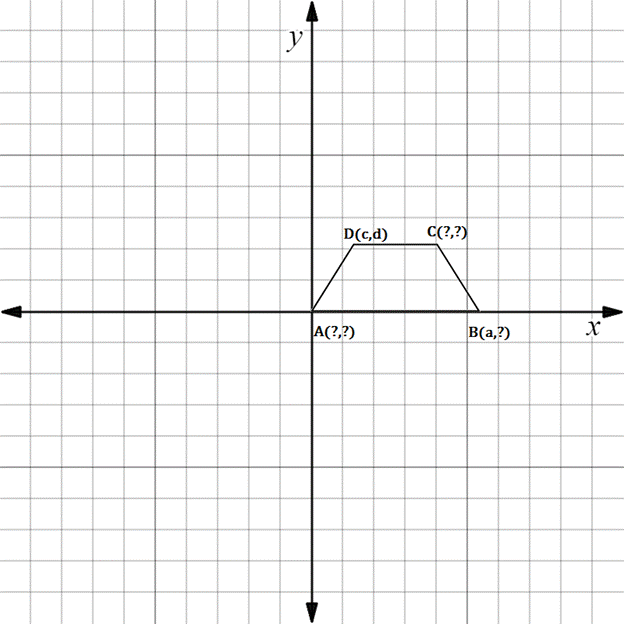Chapter 10.3, Problem 15E### Elementary Geometry for College St...

6th Edition
Daniel C. Alexander + 1 other
ISBN: 9781285195698

#### Solutions

Chapter
Section### Elementary Geometry for College St...

6th Edition
Daniel C. Alexander + 1 other
ISBN: 9781285195698
Textbook Problem
59 views

# In Exercises 11 to 16, supply the missing coordinates for the vertices, using as few variables as possible.A B C D is an isosceles trapezoid; A B - ║ D C - and A D - ≅ B C - .

To determine

To find:

The missing coordinates for the vertices.

Explanation

The given figure is shown below.

From the figure, the following observations are made.

Since, the vertex A is at the origin.

The coordinates of A0, 0.

Now, consider the vertex B.

Here, x-coordinate is given as a.

In the B vertex, observe where it lies on y-axis.

Since, it is at 0, the vertex B has the coordinates a, 0.

Consider the vertex C

### Still sussing out bartleby?

Check out a sample textbook solution.

See a sample solution

#### The Solution to Your Study Problems

Bartleby provides explanations to thousands of textbook problems written by our experts, many with advanced degrees!

Get Started

#### 13.

Mathematical Applications for the Management, Life, and Social Sciences

#### The y-coordinate of the center of mass of the region bounded by , x = 1, x = 5, y = 0 is: 4

Study Guide for Stewart's Single Variable Calculus: Early Transcendentals, 8th

#### True or False: If and , then .

Study Guide for Stewart's Multivariable Calculus, 8th